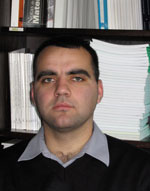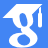Dr. Olimpiu-Flavius Pătrulescu

former member of ICTP (between 2008-2021)

This website contains information which is no longer updated

••ResearchGate Profile
•ORCID
•e-mail: flaviusolimpiu[at]yahoo.com

Ph.D. in Mathematics (2012)

## Applications of fibre contraction principle to some classes of functional integral equations

Abstract Let a<c<b real numbers, (B,|·|) a (real or complex) Banach space, H∈C([a,b]×[a,c]×B,B),K∈C([a,b]² ×B,B),g∈C([a,b],B),A:C([a,c],B)→C([a, c], B) and B:C([a,b],B)→C([a,b],B). In this paper we study the following functional integral equation, x(t)=∫_{a}^{c}H(t,s,A(x)(s))ds+∫_{a}^{t}K(t,s,B(x)(s))ds+g(t),t∈[a,b]. By…
Abstract We deal with the existence and localization of positive radial solutions for Dirichlet problems involving $$\phi$$-Laplacian operators in a ball. In particular, $$p$$-Laplacian and Minkowski-curvature equations are considered. Our…